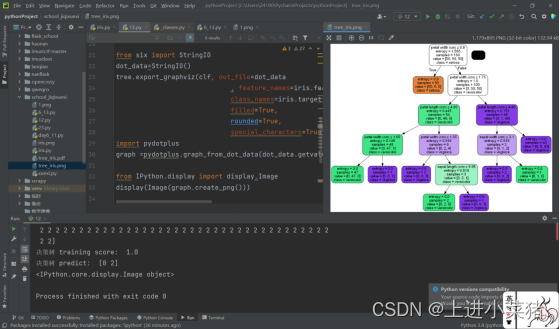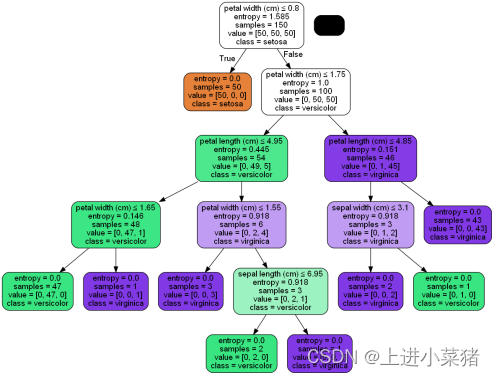# 一.前言

## 1.2 本文目的

1. 使用scikit-learn機器學習包的決策樹演算法，使用4個特徵對鳶尾花進行分類；
2. 使用ID3演算法對天氣與踢球資料集進行手工計算，手寫E(S, Temperature)、IG(S, Temperature) 、E(S, Humidity)、IG(S, Humidity)、E(S, Windy)、IG(S, Windy)的計算過程，拍照上傳、貼上圖片到實驗報告。（計算方法請參見附件PPT）
3. 使用CART演算法對天氣與踢球資料集進行手工計算，手寫Gini(S, Temperature)、Gini gain(S, Temperature) 、Gini(S, Humidity)、Gini gain(S, Humidity)、Gini(S, Windy)、Gini gain(S, Windy)的計算過程，拍照上傳、貼上圖片到實驗報告。（計算方法請參見附件PPT）
4. （選做）安裝決策樹視覺化控制元件GraphViz和pydotplus包，對鳶尾花分類結果生成決策樹視覺化圖片； # 二.實驗過程 ## 2.1 使用scikit-learn機器學習包的決策樹演算法，使用4個特徵對鳶尾花進行分類； 老規矩，先引入load_iris模組，有150組鳶尾花特徵資料，我們可以拿來進行學習特徵分類。 如下程式碼： ```from sklearn.datasets import load_iris iris = load_iris() X = iris.data y = iris.target``` 引入決策樹模組： `from sklearn import tree` 根據特徵分析，資料可分為根節點（初始節點）、中間節點和葉節點（無進一步可分離的節點）。

criterion ： string，optional（default =“gini”）

`tree.fit(X,y)` 輸出訓練得分和預測，如下程式碼： ```print("決策樹 training score: ",tree.score(X,y)) print("決策樹 predict: ",tree.predict([[7,5,2,0.5],[7.5,4,7,2]]))``` 輸出訓練得分和預測執行截圖如下：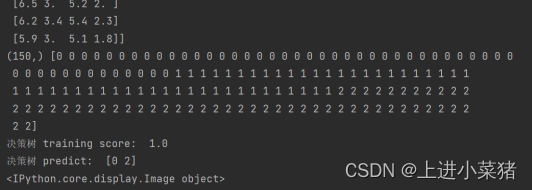## 2.2使用ID3演算法對天氣與踢球資料集進行手工計算，手寫E(S, Temperature)、IG(S, Temperature) 、E(S, Humidity)、IG(S, Humidity)、E(S, Windy)、IG(S, Windy)的計算過程

`E(S) = -[(9/14)log2(9/14) + (5/14)log2 (5/14)] = 0.94`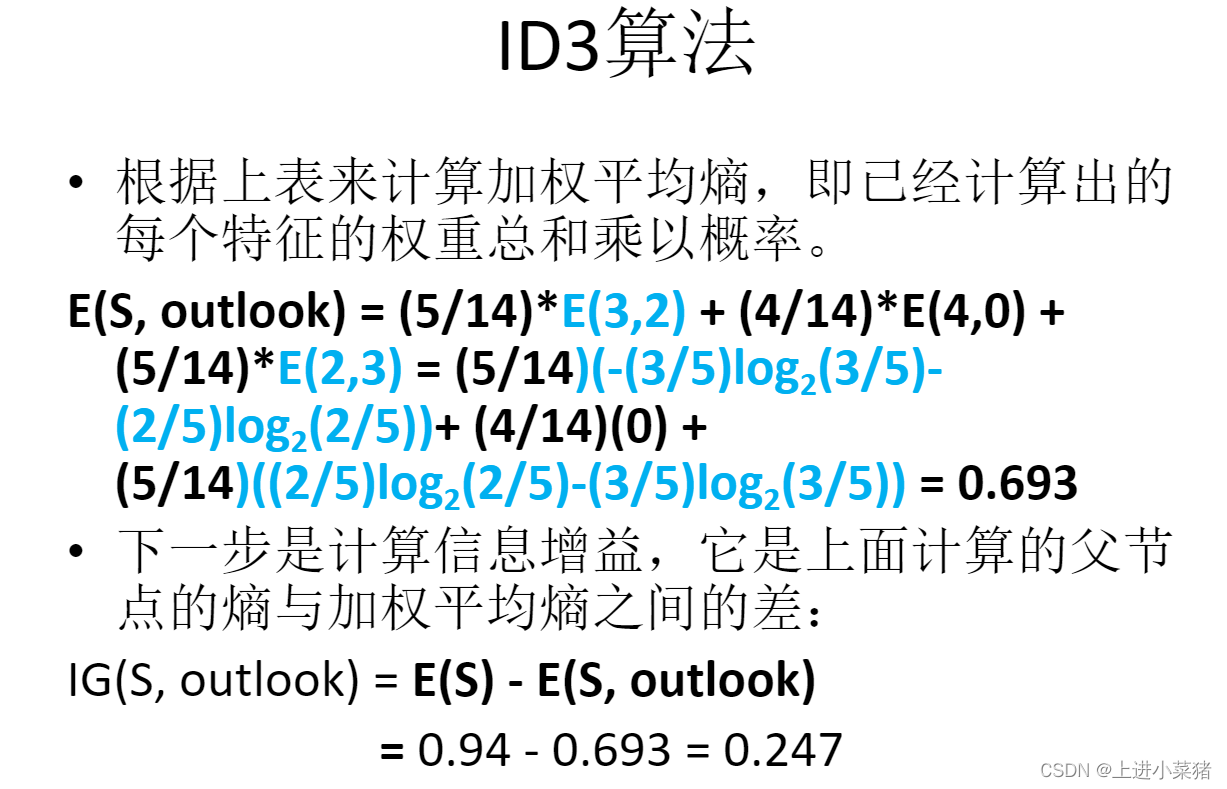E(S, Temperature)=0.911 IG(S, Temperature) =0.029 E(S, Humidity)=0.788 IG(S, Humidity)=0.152 E(S, Windy)=0.8932 IG(S, Windy)=0.048 ```

## 2.3 使用CART演算法對天氣與踢球資料集進行手工計算，手寫Gini(S, Temperature)、Gini gain(S, Temperature) 、Gini(S, Humidity)、Gini gain(S, Humidity)、Gini(S, Windy)、Gini gain(S, Windy)的計算過程

`Gini(S) = 1 - [(9/14)² + (5/14)²] = 0.4591`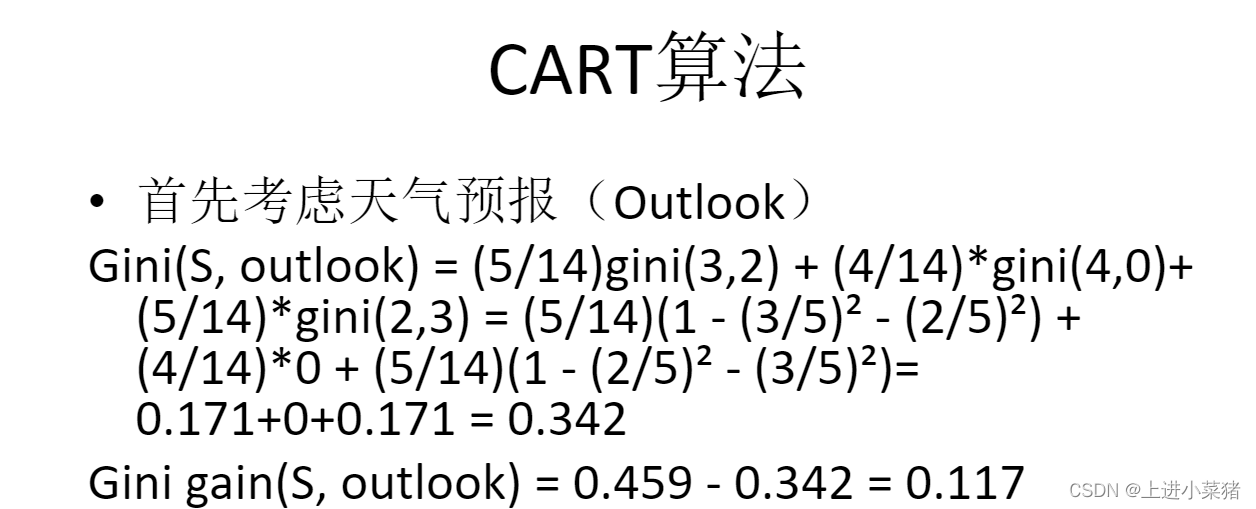本題目通過我的計算得如下結果： ```Gini(S, Temperature)=0.4405 Gini gain(S, Temperature)=0.0185 Gini(S, Humidity)=0.3674 Gini gain(S, Humidity)=0.0916 Gini(S, Windy)=0.4286 Gini gain(S, Windy)=0.0304``` 從結果來看，天氣預報（outlook）的基尼 增益為0.117，是最高的，因此我們選擇天 氣預報（outlook）作為我們的根節點。

## 2.4 安裝決策樹視覺化控制元件GraphViz和pydotplus包，對鳶尾花分類結果生成決策樹視覺化圖片；

http://www.graphviz.org/安裝一下pydotplus模組。 安裝一下os模組。 先使用os模組，指定一下路徑等等資訊。

```import os os.environ["PATH"] += os.pathsep + 'C:/Program Files/Graphviz/bin/' from six import StringIO dot_data=StringIO()``` 使用tree.export_graphviz，視覺化決策樹，如下程式碼： ```tree.export_graphviz(clf, out_file=dot_data , feature_names=iris.feature_names, class_names=iris.target_names, filled=True, rounded=True, special_characters=True)```

```使用pydotplus模組設定圖案格式等等資訊,如下程式碼： import pydotplus graph =pydotplus.graph_from_dot_data(dot_data.getvalue()) from IPython.display import display,Image display(Image(graph.create_png()))``` 指定輸出格式，輸出為pdf和png倆個 ```graph.write_png("tree_iris.png") graph.write_pdf("tree_iris.pdf")``` 我們看一下效果圖：

## 2.5 決策樹演算法之鳶尾花特徵分類視覺化效果圖